# How to Display Negative Time Value in Excel

This post will guide you how to display negative time value in Excel. How do I change the way negative times are displayed in Excel. How to show negative dates or times with a formula in Excel.

If the cell value is a negative time or date value, and the Excel will only show #### error in that cell. So how to correct this error to show the correct negative time or date.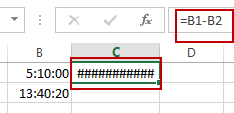## Display Negative Time Value

You can change the Excel’s Default Date System to correct this error. Just do the following steps:

#1 click File tab, and select Options from the menu list. And the Excel Options dialog will open.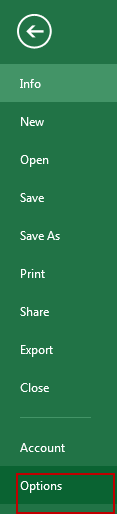#2 click Advanced from the left pane, and check Use 1904 data system under When calculating this workbook section. Click Ok button.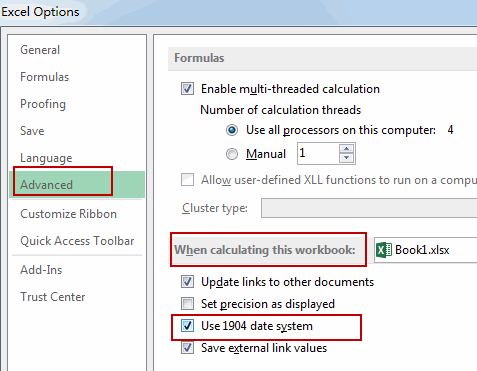#3 the negative time will be shown normally.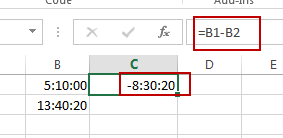## Display Negative Time Value with Formula

You can also use a formula based on the Text function, the Max function and the MIN function to achieve the result of displaying negative time value properly in Excel.

Just like this formula:

`=TEXT(MAX(\$B\$1:\$B\$2)-MIN(\$B\$1:\$B\$2),"-H::MM")`

Type this formula into a blank cell and then press Enter key in your keyboard.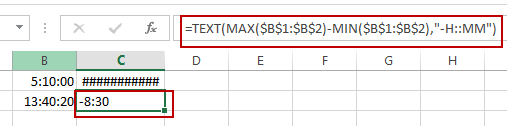You will see that a negative time value will be displayed in that cell.

The combination of the MAX function and the MIN function will subtract the earlier time from the later time, and get the positive time value. And then it will be formatted using the TEXT function so that it shows a negative time.

Note: you should know that the returned result is actually a text value, not a numeric value.

Video: Display Negative Time Value

### Related Functions

• Excel Text function
The Excel TEXT function converts a numeric value into text string with a specified format. The TEXT function is a build-in function in Microsoft Excel and it is categorized as a Text Function. The syntax of the TEXT function is as below: = TEXT (value, Format code)…
• Excel MIN function
The Excel MIN function returns the smallest numeric value from the numbers that you provided. Or returns the smallest value in the array.The MIN function is a build-in function in Microsoft Excel and it is categorized as a Statistical Function.The syntax of the MIN function is as below:= MIN(num1,[num2,…numn])….
• Excel MAX function
The Excel MAX function returns the largest numeric value from the numbers that you provided. Or returns the largest value in the array.= MAX(num1,[num2,…numn])…

Related Posts

How to Auto Fill Weekdays or Weekends in Excel

Sometimes we may want to enter a sequence of days in excel for some purpose, and we can press Ctrl+; to insert current date into cell, and then drag the cell down to attach following days into other cells, then ...

How to Change Date to The Day of Week in Excel

In excel, we can type a date properly if set cell format as ‘Date’. If there is a list includes multiple dates, is there any way to convert these dates to the days of week accordingly? Actually, there are some ...

How to Create Increment Number with Texts in Excel

Sometimes we want to fill 1,2,3,4,5… into each cell in a column, for implement this we can enter 1 in the first cell and then drag fill handle down to fill the following cells. But if there are some texts ...

How to Generate Random Time in Excel

In some special situations we may need to generate some random times in worksheet. We can enter time manually and one by one, but if we want to require a lot of random dates in excel worksheet, we will spend ...

How to Combine Text and Date into one Cell in Excel

This post will guide you how to combine text and dates in the same cell in Excel. How do I combine text and date values into one Cell in Excel 2013/2016. Combine Text and Date into One Cell Assuming that ...

How to Highlight Cell or Row If Date Is In Current Day/Week/Month in Excel

This post will guide you how to highlight cell if date is the current day or is in the current week or month in Excel. How do I highlight row if date is in current week or month with conditional ...

How to Convert Military Time to Standard Time in Excel

This post will guide you how to convert military time to standard time with a formula in Excel. How do I convert from military time 17:29:30 to regular time 5:29:30PM in Excel.  How do I Convert Standard time to military ...

How to Delete or Remove Year from a Date in Excel

This post will guide you how to delete or remove year from a given standard date in Excel. How do I remove the year from a date with a formula in Excel. Remove Year from a Date Assuming that you ...

How to Convert Date to YYYY-MM-DD format in Excel

This post will guide you how to convert the current date to a specified date format in Excel. How do I convert date to YYYY-MM-DD format with Format Cells Feature in Excel. How to convert date format to a specific ...

How to Concatenate Cells and keeping Date Format in Excel

This post will guide you how to concatenate cells and keeping data format in Excel. How do I concatenate cells and retain the certain Date format with a formula in Excel 2010/2013/2016. Concatenate Cells and Keeping Date Format Assuming that ...

Sidebar